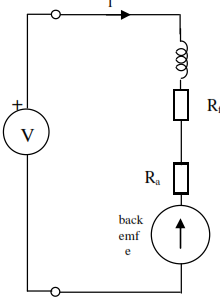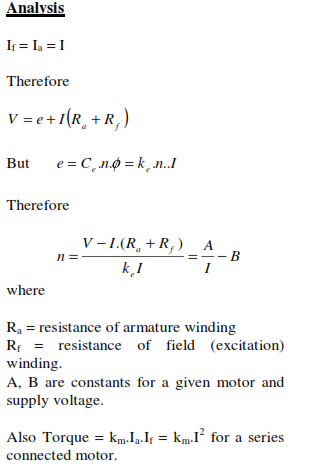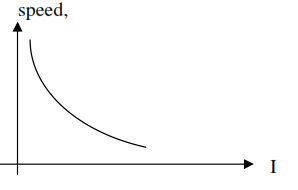Series connected motor, Electrical Engineering

Assignment Help:

In this case, the armature is connected in series with the field coils. Thus
Ia  = If = INB. At starting, the back emf is zero (because the motor is initially stationary) and hence the current is very large (limited only by the field and armature resistances). Hence the starting torque will be extremely large and if no load is applied (so that the torque requirement is small) the motor will accelerate rapidly to dangerously high speeds. A series connected motor must be started with a mechanical load attached to prevent the speed at start up rising to damaging speeds.

Note that saturation of the magnetic material in the motor can occur at high currents and then the flux generated by the field coils becomes independent of current. This causes the characteristics to become similar to that of a shunt wound motor that also has an approximately constant field flux.The series motor gives a high starting torque (proportional to 1/I2 ), 2.5 to 3 times the full load (rated) torque) but it falls rapidly as the speed increases. Applications: heavy duty, with intermittent starting under conditions where a mechanical load is always present - e.g. traction, cranes, hoists. etc

The speed of a series wound motor may be controlled by the addition of a resistor in parallel with the field coil. This diverts
some of the current away from the field coil, reducing the field strength and hence the back emf at a given speed. The additional resistor is called a 'diverter'. The speed must increase to compensate this reduction in back emf. . Hence a reduction in the diverter resistance reduces the field strength and increases the speed of the motor.

Transistor voltmeter, \ Transistor voltmeter (TVM) : a simple diagram of ...

\ Transistor voltmeter (TVM) : a simple diagram of TVM using two transistors in the bridge configuration is as shown. TVM is provided by using transistor bridge circuits. In b

Circuit symbols for mosfet, Circuit Symbols for MOSFET A range of symb...

Circuit Symbols for MOSFET A range of symbols are employed for the MOSFET. The basic design is usually a line for the channel along with the source and drain leaving it at rig

Pn-junction under bias, Q. pn-Junction under Bias? Let an external sour...

Q. pn-Junction under Bias? Let an external source be connected between the p- and n-regions, as shown in Figure (a). (b) shows the circuit representation of the pn-junction or

Analysis of financial plans, Complete the financial reporting for each peri...

Complete the financial reporting for each period and develop recommendations using the templates provided. Procedure 1.  Read the case study. 2.  Complete the financial re

Voltage, the relation between power

the relation between power

Circuit with capacitive load Capacitors which obey the relationship of equation are linear capacitors, since the potential difference among the conductive surfaces is linearly

Determine the transfer function in notch network, Q. The twin-tee or notch ...

Q. The twin-tee or notch network shown in Figure is often used to obtain band-reject characteristics. (a) Determine the transfer function V 2 /I 1 . (b) Find the angular freq

Develop a block diagram of an asynchronous decade counter, Q. Counting to m...

Q. Counting to moduli other than 2 is a frequent requirement, the most common being to count through the binary-coded decimal (BCD) 8421 sequence. All that is required is a four-st

Grid connected hybrid pv-wind power system, Hi there, My project title is g...

Hi there, My project title is grid connected hybrid pv-wind power system. For this topic, i need a specification of the system. For this system a matlab and homer software simulati

Buck converter - power supplies , Buck Converter In buck  converters th...

Buck Converter In buck  converters the output  voltage is  always  less than the  input  voltage. The basic  circuit  of buck converter is shown  in figure. The operation of th